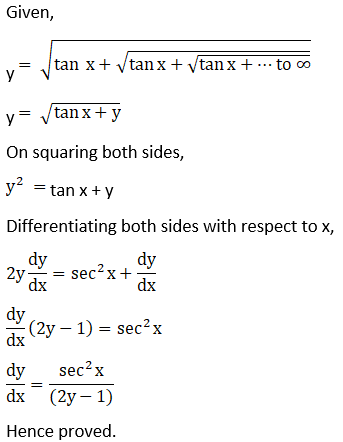# RD Sharma Solutions For Class 12 Maths Exercise 11.6 Chapter 11 Differentiation

RD Sharma Solutions for Class 12 Maths Exercise 11.6 Chapter 11 Differentiation is provided here. This exercise deals with the differentiation of an infinite series. Infinite series are defined as the limit of the infinite sequence of partial sums. Prior to this exercise, more number of detailed illustrations are provided, which explains the steps involved in differentiating the given infinite series.

Students can access exercise-wise solutions in PDF format to solve problems of RD Sharma textbook easily. These solutions improve the skill of managing time, which is important from the exam point of view. Students who have doubts regarding these concepts can access RD Sharma Solutions for Class 12 Maths Chapter 11 Exercise 11.6 from the below provided links.

## RD Sharma Solutions For Class 12 Chapter 11 Exercise 11.6:-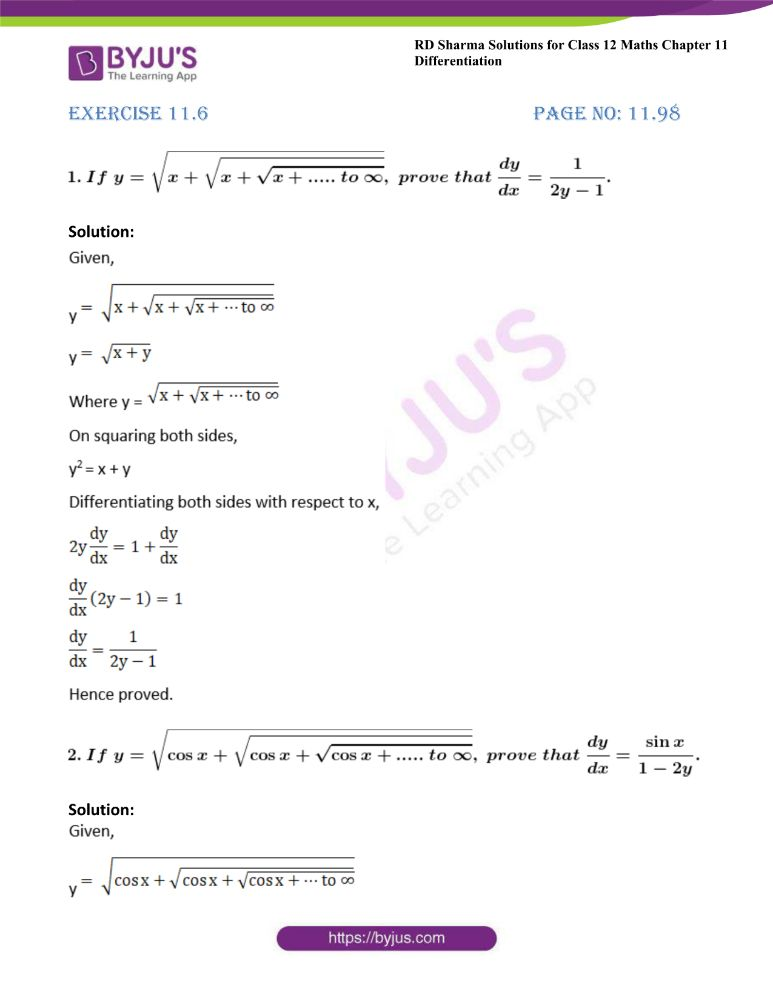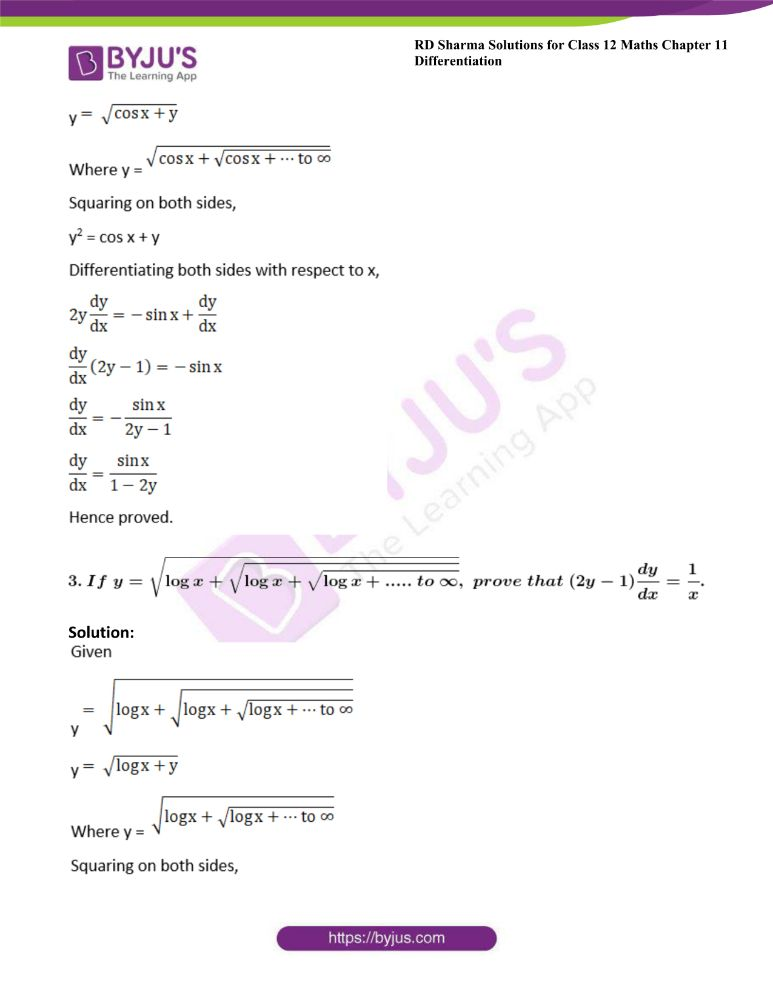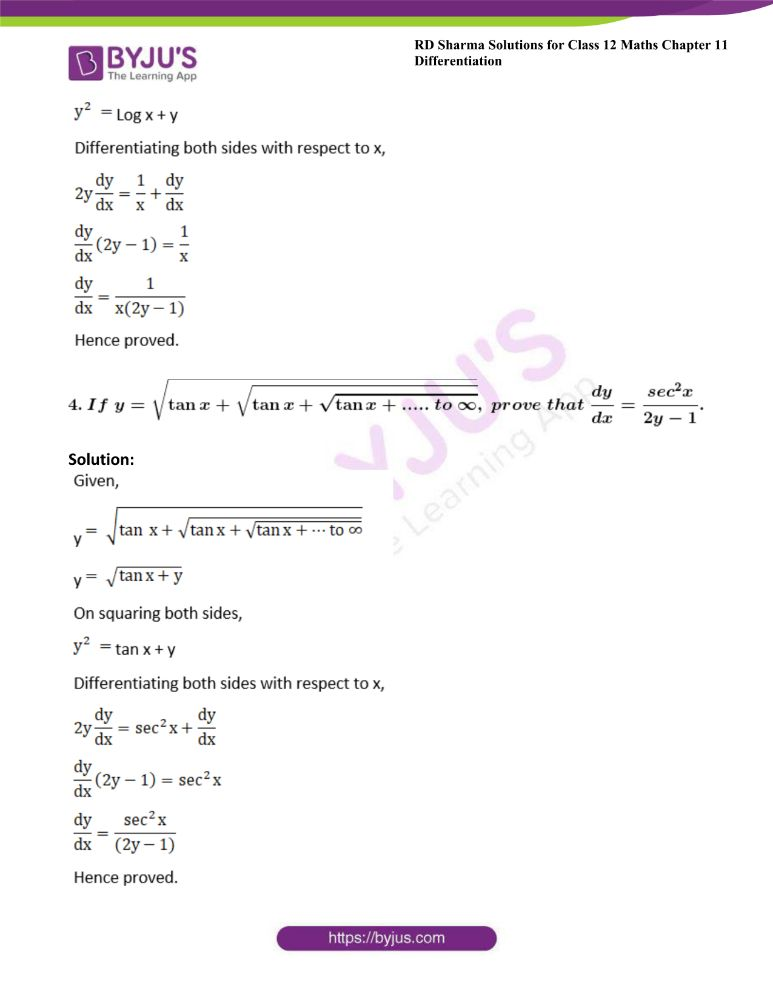### Access other exercises of RD Sharma Solutions For Class 12 Chapter 11 – Differentiation

Exercise 11.1 Solutions

Exercise 11.2 Solutions

Exercise 11.3 Solutions

Exercise 11.4 Solutions

Exercise 11.5 Solutions

Exercise 11.7 Solutions

Exercise 11.8 Solutions

### Access answers to Maths RD Sharma Solutions For Class 12 Chapter 11 – Differentiation Exercise 11.6

Exercise 11.6 Page No: 11.98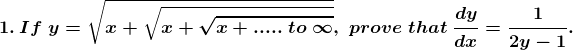Solution: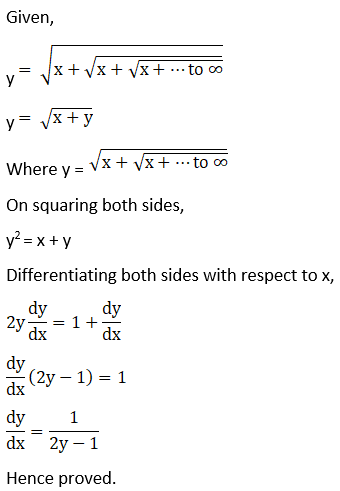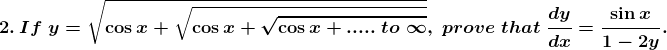Solution: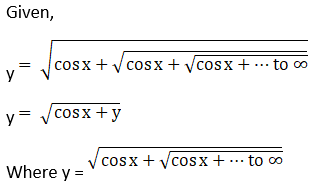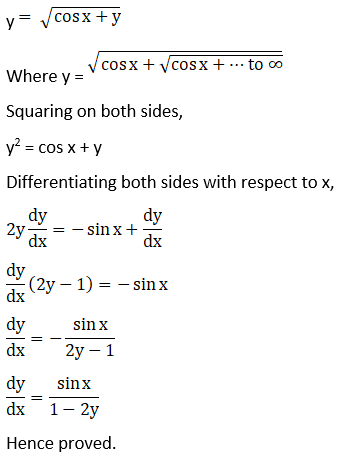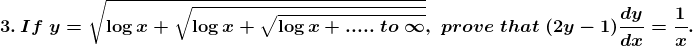Solution: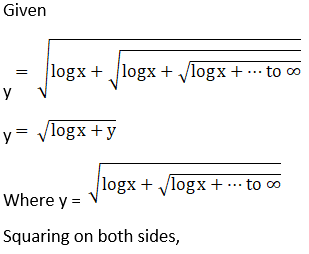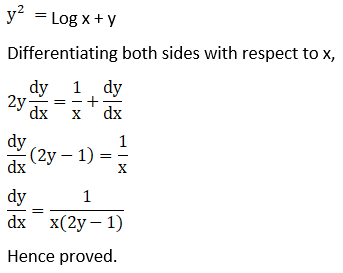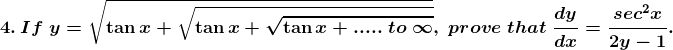Solution: